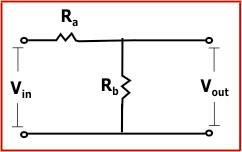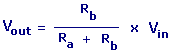Voltage Divider Calculator

Enter any three of the following values and then click calculate button.Vin = Input Voltage Vout = Output VoltageVin: Volts Ra: Ohms Rb: Ohms Vout: Volts

 Web www.calculatoredge.com
 A voltage divider (also known as a potential divider) consists of two resistances Ra and Rb connected in series across a supply voltage Vin. The supply voltage is divided between the two resistances to give an output voltage Vout which is the voltage across Rb.

This configuration is known as a voltage divider because it divides the input voltage into two parts.LINKSDISCLAIMERCONTACT US